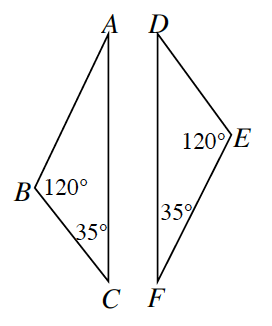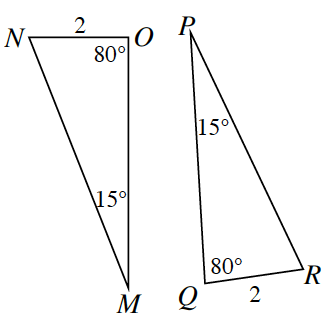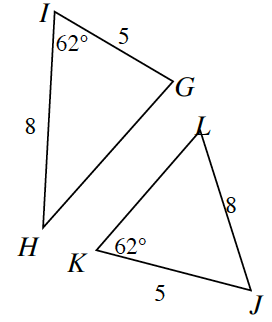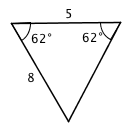Home > CCG > Chapter 6 > Lesson 6.2.2 > Problem6-63

6-63.

Determine if each pair of triangles below are congruent, similar but not congruent, or if they are neither. If they are congruent, organize your reasoning into a flowchart. Remember that the triangles below not be drawn to scale.

Use the triangle congruence conditions explained in the Math Notes box in Lesson 6.1.4.

1.Similar by $\text{AA}\sim$.

1.$ΔMON≅ΔPQR$

$\text{AAS}≅$ or $\text{ASA}≅$

1.For the two triangles to be congruent, they should look like the diagram shown below. Is this triangle possible? If the triangles are not congruent, is it possible for them to be similar?